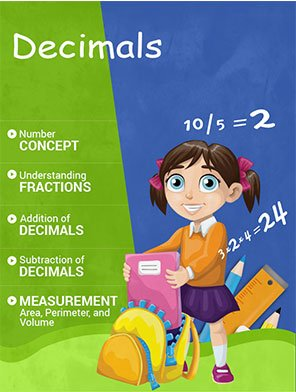\$7.00

## MATH DECIMALS WORKSHEETS

A decimal is a fraction having denominator as the power of 10. So, students start decimal concept with converting fractions into decimals and vice-versa. Students practice more problems related to four operations on fractions and decimals than previous fraction concept. Then, they will get knowledge of adding, subtracting, multiplying and dividing decimals in this section.

Worksheet # Topics/Curriculum # of Pages 2pg Sample Add to cart
Unit-1 Conversion of Fractions and Decimals 8 pages\$1.00

Unit-2 Fractions and Decimals Operation 8 pages\$1.00

Unit-3 Simplifying Fractions and Decimals 8 pages\$1.00

Unit-4 More Problems on Simplifying Fractions and Decimals 8 pages\$1.00

Unit-5 Four Operations on Fractions and Decimals 8 pages\$1.00

Unit-6 More Problems on Four Operations on Fractions and Decimals 8 pages\$1.00

Unit-7 Addition of Decimals 8 pages\$1.00

Unit-8 Subtraction of Decimals 8 pages\$1.00

Unit-9 Multiplication of Decimals 8 pages\$1.00

Unit-10 Division of Decimals 8 pages\$1.00